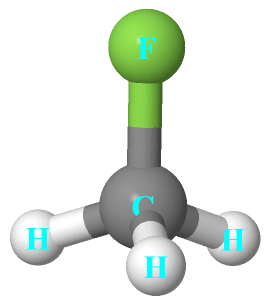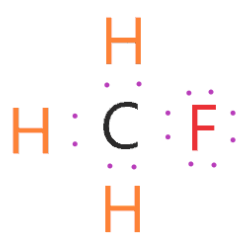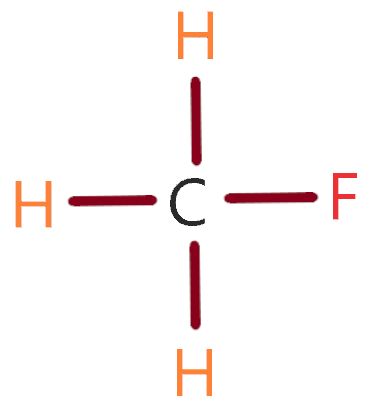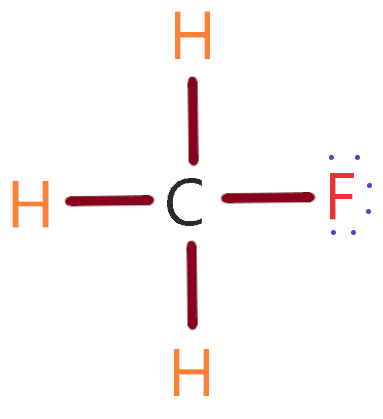# Fluromethane (CH3F) lewis dot structure, Molecular geometry, polarity, dipole moment, hybridization

Home  > Chemistry Article > CH3F lewis structure and its molecular geometryCH3F is a non-toxic and liquefiable gas also known as Fluoromethane or methyl fluoride. It is a colorless and flammable gas.

We will learn in this article about important aspects of methyl fluoride like CH3F lewis structure, molecular geometry, is CH3F polar or non-polar, hybridization, and its dipole moment.

CH3F is used as an etching gas in plasma etch reactors which is useful in the semiconductor manufacturing process. This gas is heavier than air. Mostly is used as a refrigerant. It has an ether-like odor at high concentrations.

Fluoromethane belongs to the hydrofluorocarbon family and is the lowest mass member in their family. CH3F becomes a narcotic in high concentrations.

CH3F is not as destructive or harmful as CH3Cl to the ozone layer because it only contains carbon, hydrogen, and fluorine. And chlorine is somewhat harmful to the ozone layer.

Properties of CH3F

• It molar mass is 34.03 g/mol.
• The density of CH3F is 1.4397 g/L and 0.557 g/cm³ at saturated pressure and temperature.
• The melting point of CH3F is -137.8ºC and the Boiling point is -78.4ºC.
• Its vapor pressure is 3.3 MPa.
• Its IUPAC name is Fluoromethane.
 Name of Molecule Fluoromethane Chemical formula CH3F Molecular geometry of CH3F Tetrahedral Electron geometry of CH3F Tetrahedral Hybridization Sp³ Bond angle 109.5º Total Valence electron for CH3F 14 The formal charge of CH3F 0
Page Contents

## How to draw lewis structure of CH3F (Fluoromethane)?

CH3F lewis’s structure contains one carbon attached with three hydrogen atoms and one fluorine atom. Being the least electronegative, carbon is the central atom in CH3F lewis’s structure.

There is a total of 4 bonded pairs and 3 lone pairs present in the CH3F lewis dot structure.

## To draw the lewis dot structure of CH3F follows some simple steps

Step 1: In the first step, Count all valence electrons present in CH3F. For finding valence electrons simply remember the periodic table group.

⇒ As Hydrogen lies in the first group, so, valence electron of hydrogen is = 1

⇒ Similarly, Carbon is present in the 14th group, so, the valence electron of carbon is = 4

⇒ And Fluorine is present in the 17th group, so, its valence electron is = 7

∴ Total number of valence electrons that is available for drawing the lewis diagram of CH3F = 4 + 1*3 + 7 = 14 valence electrons.Step 2: Now find the least electronegativity atoms present in CH3F and after it put that atom at the center. Clearly, Carbon is the least electronegative atom because it belongs 14th group, and fluorine belongs to the 17th group, and electronegativity increases from left to right in the periodic table.

“Hydrogen atom always goes outside in lewis diagram”.

Hence carbon is the least electronegative atom and put it at the center in the lewis diagram and other atoms spaced evenly around it.Step 3: After these, Structured all atoms in CH3F by connecting the outer atoms to the central atom.Step 4: Now complete the octet rule for every atom present in the CH3F molecule. First, follow the octet rule for outer atoms then moved to the central atom.

As hydrogen atoms are happy with only two electrons that are already present in a single bond, So, their outer shell is full.

Put 6 electrons around fluorine and complete its octet rule as fluorine atom already shared 2 electrons with the help of a single bond.

Carbon central atom shared 8 electrons with the help of 4 single bonds as every single bond contains 2 electrons, so, it also completes the octet rule.

###Lewis dot structure of CH3F

So, all the atoms in the above structure have completed the octet comfortably, hence, we got our best lewis structure of CH3F.

## Find the Molecular geometry of CH3F and its hybridization

With the help of the VSEPR (Valence shell electron pair repulsion) theory, we will determine the molecular geometry of CH3F and its hybridization.

To find the shape of any molecule simply remember these conditions given below:

1: If the Total Valence electrons of a given molecule are less or equal to 8 then divide it by 2.

⇒ V.E. ≤ 8 then divide by 2

2. If the valence electron of a given molecule lies between 8 to 56 then divide it by 8.

⇒ 8 ≤ V.E. ≤ 56 then divide with 8.

3. If the valence electron of a given molecule is greater than 56 then divide it by 18.

⇒ V.E. > 56 then divide with 18.

∴ Now CH3F has a 14 total valence electron so divide it with 8

⇒ 14 ÷ 8

After dividing we got 6 remainder and 1 quotient so again apply the valence electron rule V.E. ≤ 8 and divide it with 2. After that, we got 3 quotients and zero remainders.

So, add both Quotients we got

⇒ 1 + 3 = 4

Now 4 orbital means CH3F has Sp³ hybridization. Carbon and Fluorine has also Sp³ hybridization and Hydrogen atoms are unable to hybridize so leave it as usual.

The molecular shape or electron geometry of CH3F is Tetrahedral because three hydrogens and one fluorine atom are bonded to the carbon central atom and no lone pair is present on the central atom that makes its shape look like tetrahedral.

VSEPR notation is AX4 or AX4E0 for these types of molecules.### CH3F Molecular geometry or shape

CH3F has a bond angle of 109.5 degrees because it has a tetrahedral structure and also the presence of a lone pair causes the reduction of the bond angle in the CH3F molecule.

## Polarity: Is CH3F polar or non-polar and its Dipole moment

CH3F has non zero dipole moment because the Fluorine atom has more electronegativity than carbon and hydrogen. So, fluorine attracts a partial negative charge and other atoms (Carbon or Hydrogen) attract a partial positive charge which causes CH3F non-zero dipole moment. And this molecule(CH3F) has a dipole moment of 1.8 D.

As dipole moment of CH3F has non-zero and it has a molecular structure like tetrahedral. Also, due to the attraction of negative charge by fluorine, it behaves like negative poles and positive charge attracted by carbon or hydrogen behaves like a positive pole.

Due to the difference of these poles dipole moment cannot be canceled. Hence, all these factors cause to CH3F becomes polar in nature.

The polarity of CH3F depends on the following factors:-

1. Electronegativity:

It directly influences the polarity nature of any atom or molecules. Because polarity is directly proportional to the difference of electronegativity created by atoms or molecules.

So, in this CH3F molecule case, Fluorine has a greater electronegativity (3.98) and Carbon has a 2.6 electronegativity. As more electronegativity shows that a molecule or atom has more tendency to attract the bonded pair towards it than fewer electronegativity molecules.

Both Fluorine and Carbon attract some negative or positive charge which causes asymmetric charges distribution around atoms. Hence it strengthens the polar nature of CH3F (Fluoromethane).

2. Geometry or Shape:

With the help of CH3F lewis’s structure or electron structure we can guess the idea that is CH3F polar or non-polar.

CH3F has a tetrahedral structure and asymmetric in nature. And in chemistry almost all molecule which has asymmetric structure they have the tendency to become polar. So, with the help of molecular geometry or shape, we can easily guess whether the given molecule is polar or non-polar.

3. Dipole moment:

This factor also directly influences the polarity of molecules. As greater the dipole moment of the molecule more is the polar nature of that molecule. The dipole moment of CH3F is 1.8D.

Also, the CH3F shape is asymmetric therefore dipole moment of this molecule does not cancel each other.

In mathematical term, we can express dipole moment as D = Q × R

Dipole moment formula =  charge on the atoms * the distance between them

## Summary

A quick overview of CH3F lewis’s structure and molecular geometry about what we have learned in this article.

• CH3F is non-toxic gas which is also known as Fluoromethane or methyl fluoride.
• The molecular shape of CH3F is tetrahedral.
• The hybridization of CH3F is Sp³.
• The dipole moment of CH3F is 1.8D.
• The bond angle of CH3F is 109.5 degrees.
• CH3F is polar due to its asymmetric shape.
• The total valence electron in CH3F is 14.

## FAQ

### How many lone pairs are in the CH3F lewis structure?

It has three lone pairs as from the lewis structure of CH3F, we can easily see that the Fluorine atom has 6 electrons which are not shared with any of the covalent bonds. Hence, these 6 electrons make 3 lone pairs in the fluoromethane lewis structure.

### What intermolecular forces are present in CH3F molecular geometry?

London dispersion force is present in CH3F molecular geometry. Because due to the electronegativity difference of carbon and fluorine atoms it formed a dipole which causes negative charges and positive charges attracted by different atoms present in CH3F.

As London dispersion force is caused by positive nuclei and electrons. Hence this force is present in CH3F.

### How many valence electrons and non-shared electrons are in the CH3F lewis dot structure?

A total of 14 valence electrons and 6 non-shared electrons are present around the fluorine.

### why does CH3F not have hydrogen bonding?

As we saw in the Lewis structure of CH3F(Fluoromethane), Fluorine is bonded with carbon central atom, and Hydrogen is attached only with Carbon, not with Fluorine. So, in order to the occurrence of hydrogen bond Fluorine must be attached with hydrogen. That is why CH3F does not have hydrogen bonding.
##### Subscribe to Blog via Email

Join 2 other subscribers

Share it...